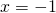# Solution assignment 08 Equations with fractions

### Assignment 8

Solve: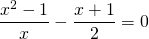### Solution

Cross-multiplication yields: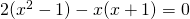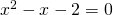The left-hand side of this equation can be factorized: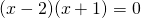and thus the solutions are: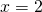or